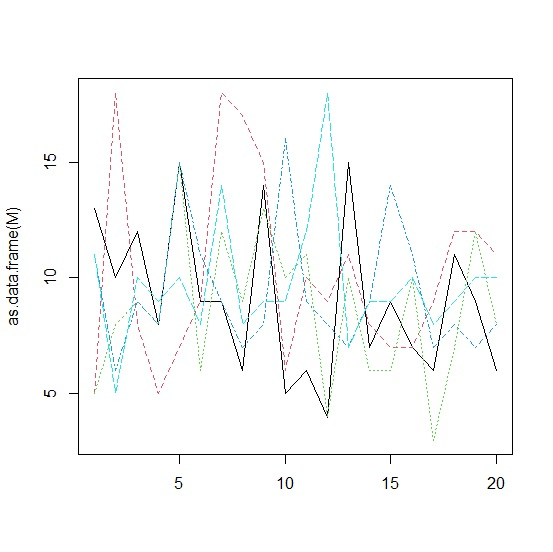# How to plot matrix columns as lines in base R?

To plot matrix columns as lines in base R, we can use matplot function but we need to read the matrix as a data frame using as.data.frame function and for creating lines the type argument will be used. For example, if we have a matrix called M then the columns of M can be plotted as lines using the command matplot(as.data.frame(M),type="l").

Consider the below data frame −

## Example

Live Demo

M<-matrix(rpois(100,10),nrow=20)
M

## Output

    [,1] [,2] [,3] [,4] [,5]
[1,] 13    5    5   11   11
[2,] 10   18    8    6    5
[3,] 12    8    9    9   10
[4,]  8    5    8    8    9
[5,] 15    7   15   15   10
[6,]  9    9    6   11    8
[7,]  9   18   12    9   14
[8,]  6   17    9    7    8
[9,] 14   15   13    8    9
[10,] 5    6   10   16    9
[11,] 6   10   11    9   12
[12,] 4    9    4    8   18
[13,] 15  11   10    7    7
[14,]  7   8    6    9    9
[15,]  9   7    6   14    9
[16,]  7   7   10   11   10
[17,]  6   9    3    7    8
[18,] 11  12    7    8    9
[19,]  9  12   12    7   10
[20,]  6  11    8    8   10

Creating a plot of columns of matrix M with lines −

## Example

matplot(as.data.frame(M),type="l")

## Output